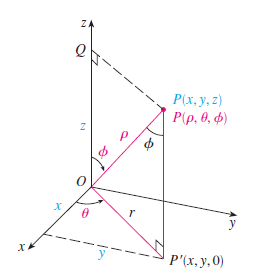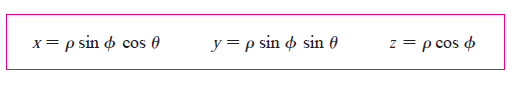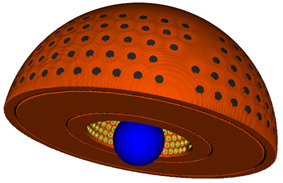# How to shift before rotating the geometry

• Hi everyone, I want to translate geometry and rotate a geometry in G4PVPlacement but it seems to do the rotation before the displacement doing this is quite difficult for me. I want to do the translation before I do the rotation, is it possible? If yes, please help me.
• I would like someone to explain to me about G4RotationMatrix, When rotating an angle around the X or Y or Z axis, how is the rotation direction, where does the rotation angle start?

Hello,
A positive rotation angle is a clockwise rotation around an axis if you look from the positive direction of the axis e.g. :

``````  //Create a Rotation Matrix
G4RotationMatrix* Rotation = new G4RotationMatrix();
Rotation->rotateX(0*deg);
Rotation->rotateY(20*deg);
Rotation->rotateZ(0*deg);
``````

will rotate the object it is assigned to 20° clockwise around its internal y-axis if look from above.
Of course one could also say it rotated the object 20° counterclockwise if you look from the negative side of the axis.

An object is not rotated around the world volume axes or the mother volumes axes (as far as i know).
Every object has in internal coordinates system; doesn’t matter where you place it. Rotations are relative to this internal coordinate system.
So it doesn’t matter if you translate an object and rotate it or if you rotate first and then translate it. The outcome will be the same.

What are you trying to do that you need to translate it first and then rotate it?

Regards

Dear Sir,
Thank you for your answer. It’s very useful for me. I’m a newbie in Geant4 and I don’t have more experience. For a long time, I have worked with MCNP. Maybe you know, MCNP only uses the global coordinate. So I thought that the rotation and translation were performed with the same coordinate. With the MCNP, I just placed the volume at a specific location and rotated that easily.
I will try again right away. Thank you one more time.

Dear Sir,
I have another question about the rotation angle. If I rotate Theta-angle around OZ-axis, the Theta=0 deg is on OX-axis, right? Similarly, if Phi is rotated around the OX axis, then Phi = 0 deg will lie on the OZ axis, right? Are these mathematical conventions true in Geant4 or not?Hello again,
I did not encounter any case where Geant4 mathematical conventions differ from ‘real world’ mathematical conventions… so yes, i guess they are true.

I don’t understand why you are using spherical coordinates… Is this still relevant for understanding the G4RotationMatrix?

Dear Sir,
Thank you for your kindness. I think that I can explain some things about my project. In the attached figure, that is my geometry included sphere block and 201 source channels. So, I created the channel. That’s why I used spherical coordinates to calculate the position to place them. And I thought that the G4RotationMatrix() worked as the mathematical conventions.